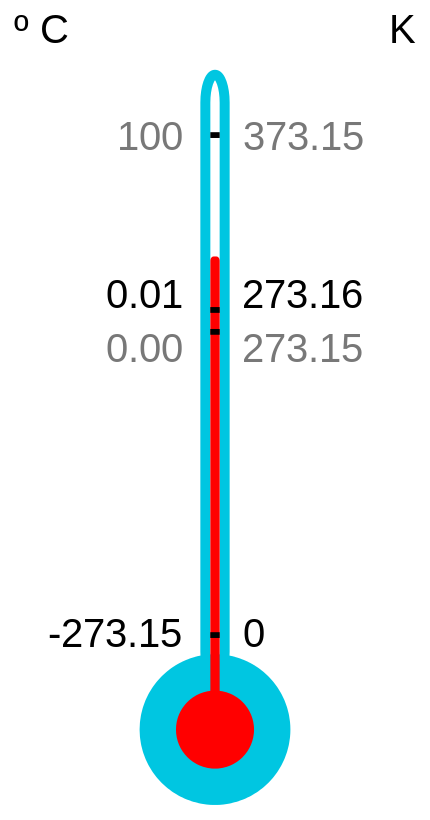# At what temperature in degrees Kelvin does water boil?

The Kelvin system of temperature measurement takes its base point as absolute zero, which is commonly taken to be -273.15 degrees Celsius, or -459.67 degrees Fahrenheit. Absolute zero is Kelvin is known as 0 degrees K. The scale in Kelvin works it way upward from 0 K.As you can see, it is quite simple to convert temperatures in Celsius to Kelvin. All you need to do is add 273.15 to the number in degrees Celsius. To find out the freezing point of water in K, the sum is 0 (degrees C at which water freezes) + 273.15 = 273.15K A hot sunny day might reach 32C; in K this would be 32 + 273.15 = 305.15K. Therefore, the boiling point of water would be 100 = 273.15 = 373.15K. To convert Fahrenheit to Kelvin is a lot more complicated, so click here for help.

The Invention of Degrees Kelvin

The Kelvin system was invented in 1848 by William Thomson, 1st Baron of Kelvin, and an engineer at Glasgow University. Also known for his work on the transatlantic telegraph system and the mariner's compass, Thomson also discovered the coldest possible temperature that could be achieved (though he gave it at -273C, rather than -273.15C, at the time) and proposed a new measurement since using this as its base point.

This film explains more about the science of absolute zero:

thanked the writer.